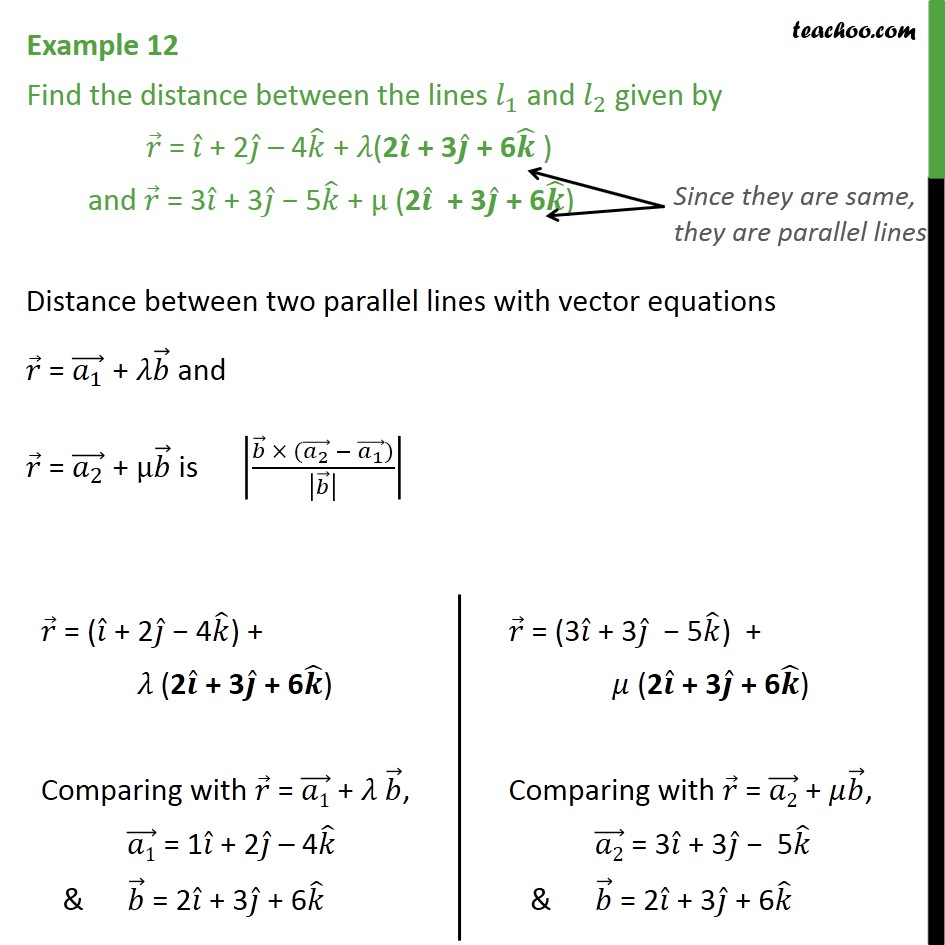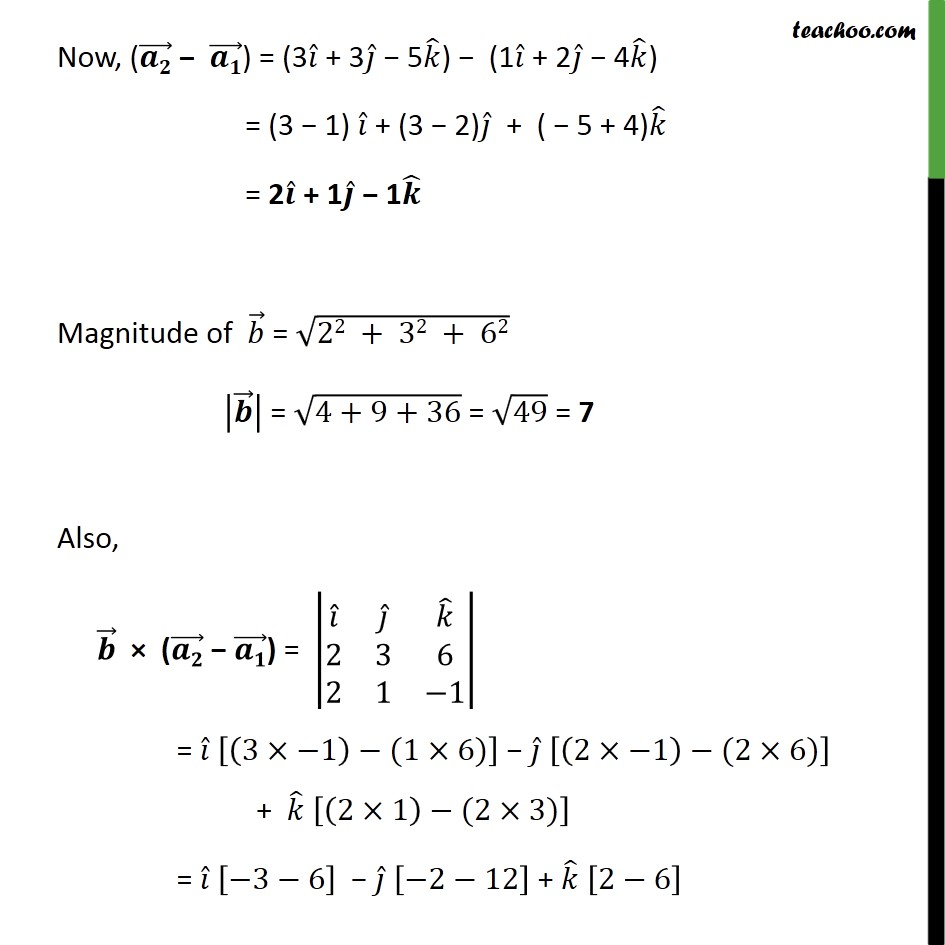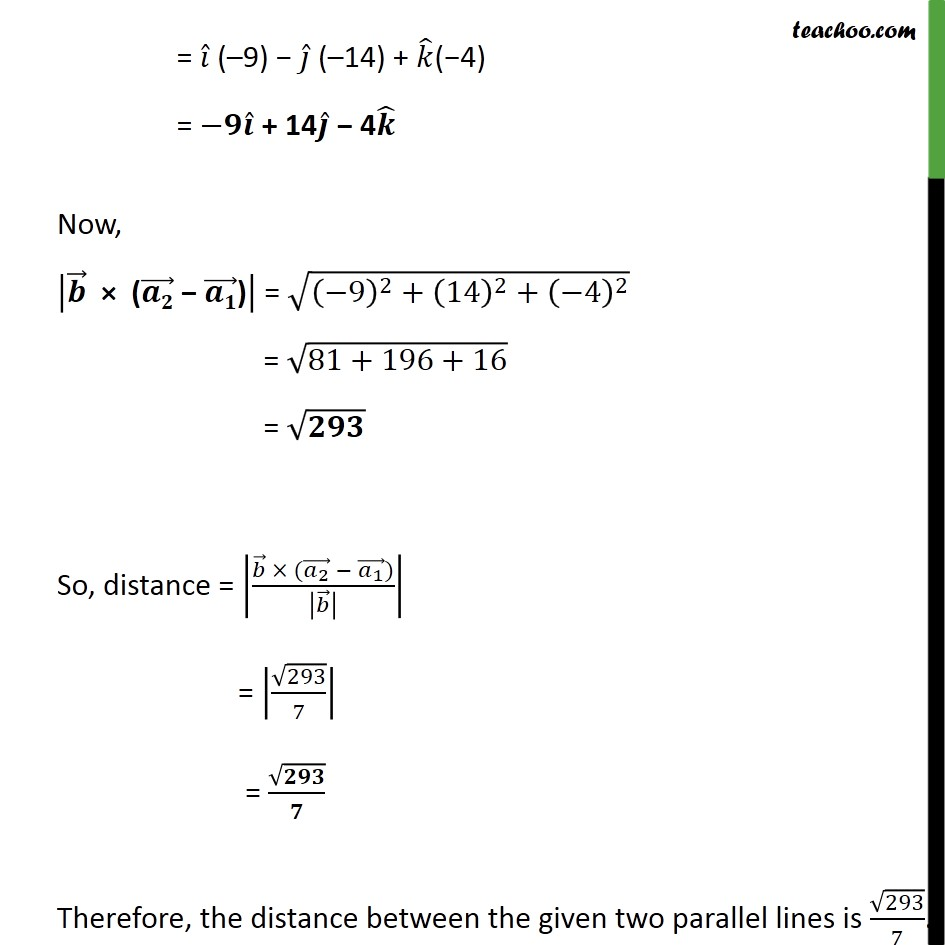1. Chapter 11 Class 12 Three Dimensional Geometry
2. Concept wise
3. Shortest distance between two parallel lines

Transcript

Example 12 Find the distance between the lines 𝑙﷮1﷯ and 𝑙﷮2﷯ given by 𝑟﷯ = 𝑖﷯ + 2 𝑗﷯ – 4 𝑘﷯ + 𝜆(2 𝒊﷯ + 3 𝒋﷯ + 6 𝒌﷯ ) and 𝑟﷯ = 3 𝑖﷯ + 3 𝑗﷯ − 5 𝑘﷯ + μ (2 𝒊﷯ + 3 𝒋﷯ + 6 𝒌﷯) Distance between two parallel lines with vector equations 𝑟﷯ = 𝑎﷮1﷯﷯ + 𝜆 𝑏﷯ and 𝑟﷯ = 𝑎﷮2﷯﷯ + μ 𝑏﷯ is 𝑏﷯ × ( 𝑎﷮2﷯﷯ − 𝑎﷮1﷯﷯)﷮ 𝑏﷯﷯﷯﷯ Now, ( 𝒂𝟐﷯ − 𝒂𝟏﷯) = (3 𝑖﷯ + 3 𝑗﷯ − 5 𝑘﷯) − (1 𝑖﷯ + 2 𝑗﷯ − 4 𝑘﷯) = (3 − 1) 𝑖﷯ + (3 − 2) 𝑗﷯ + ( − 5 + 4) 𝑘﷯ = 2 𝒊﷯ + 1 𝒋﷯ − 1 𝒌﷯ Magnitude of 𝑏﷯ = ﷮22 + 32 + 62﷯ 𝒃﷯﷯ = ﷮4+9+36﷯ = ﷮49﷯ = 7 Also, 𝒃﷯ × ( 𝒂𝟐﷯ − 𝒂𝟏﷯) = 𝑖﷯﷮ 𝑗﷯﷮ 𝑘﷯﷮2﷮3﷮6﷮2﷮1﷮−1﷯﷯ = 𝑖﷯ 3×−1﷯−(1×6)﷯ − 𝑗﷯ 2×−1﷯−(2×6)﷯ + 𝑘﷯ 2×1﷯−(2×3)﷯ = 𝑖﷯ −3−6﷯ − 𝑗﷯ −2−12﷯ + 𝑘﷯ 2−6﷯ = 𝑖﷯ (–9) − 𝑗﷯ (–14) + 𝑘﷯(−4) = −𝟗 𝒊﷯ + 14 𝒋﷯ − 4 𝒌﷯ Now, 𝒃﷯ × ( 𝒂𝟐﷯ − 𝒂𝟏﷯)﷯ = ﷮ −9﷯﷮2﷯+ 14﷯﷮2﷯+ −4﷯﷮2﷯﷯ = ﷮81+196+16﷯ = ﷮𝟐𝟗𝟑﷯ So, distance = 𝑏﷯ × ( 𝑎﷮2﷯﷯ − 𝑎﷮1﷯﷯)﷮ 𝑏﷯﷯﷯﷯ = ﷮293﷯﷮7﷯﷯ = ﷮𝟐𝟗𝟑﷯﷮𝟕﷯ Therefore, the distance between the given two parallel lines is ﷮293﷯﷮7﷯.

Shortest distance between two parallel lines

About the AuthorDavneet Singh
Davneet Singh is a graduate from Indian Institute of Technology, Kanpur. He has been teaching from the past 9 years. He provides courses for Maths and Science at Teachoo.